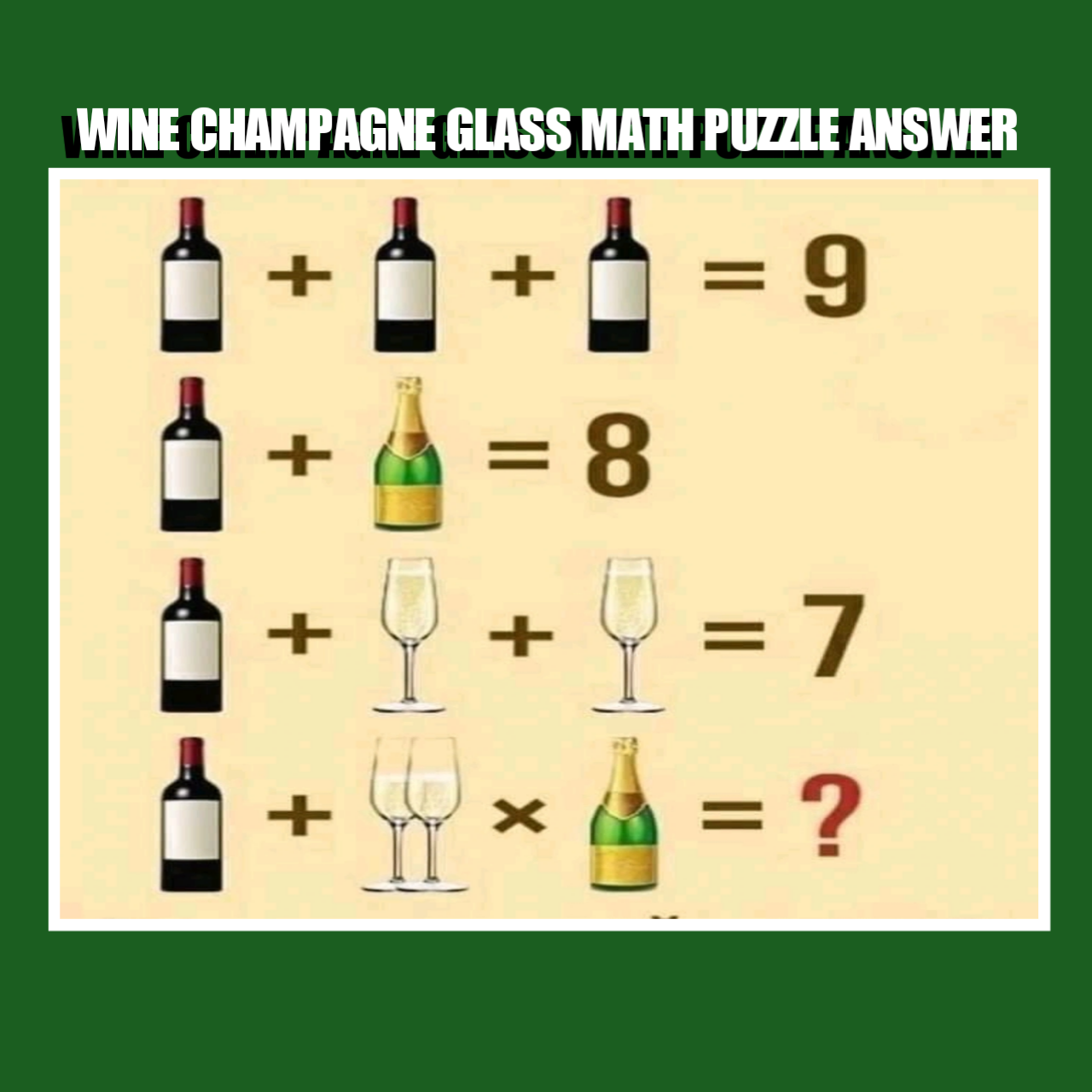0

# Wine champagne glass math puzzle answerWine champagne glass math puzzle answer

This is one of those math  puzzles that keeps many people scratching their heads on the internet, especially on Facebook.  When the Puzzle was published on Facebook, many people solved it and had different opinions and answers. Today I will give you the correct answer to the puzzle with solving the details.

Wine + 2half glass × Champagne = ?

The correct answer would be 13, because in the math puzzle, there are two half full glasses of wine and not a full glass in the final equation. If you don't apply BODMAS correctly, you will not also get the correct answer.

Check the solution below.

Here's the puzzle extraction:

If

Wine  + wine + wine bottle = 9,

Wine + champagne  bottle = 8,

Wine + full glass + full glass = 7

then,

Wine + 2 half full glass  × champagne = ?

Due to the Corona various pandemic, many people isolate themselves because of lockdowns and to avoid contracting or spreading the deadly disease.

Isolation can lead to boredom,  one way to refresh the brain, relax and get busy is  by solving Math puzzles and Quizzes.

Many game lovers like solving math puzzles suduku, quizzes like this one to refresh their brain

I will solve each equation and then substitute answers in the final equation and simplify using BODMAS

Equation one

3 wine bottles=9

Implies A wine bottle= 9÷3,

Wine bottle = 3.

Equation two

3 + champagne bottle = 8

Implies Champagne bottle = 8 minus 3

champagne bottle= 5.

Equation three

3 + full glass+ full glass = 7

2full glasses = 7 minus 3

2full glasses = 4

Full glass = 4 ÷ 2

Full glass = 2.

Here's where the trick in the drink math puzzle is

If full glass = 2, then half full glass = 1.

Therefore, the value of the variables are as Thus:

Wine= 3

Champagne bottle = 5

Full glass = 2

Half full glass  = 1

Final equation

I will substitute the values of the variables above in the final equation below and simplify using BODMAS.

Wine + 2half glass × Champagne = ?

3 + 2(1) × 5=?

3 + 2 × 5 =?

3 + 10

13  = ?

So the correct answer to the tricky  math Puzzle Half Wine + 2half glass × Champagne = ?

Is

= 13.

Conclusion.

Applying basic mathematics like Solving equations, Making subject of the formula, Substitution, Applying BODMAS will always give you the correct answer.

Do you have another way to arrive at this Answer? Share with us.

Don't forget to test your friends, family members and loved ones by sharing the puzzle.

Tags